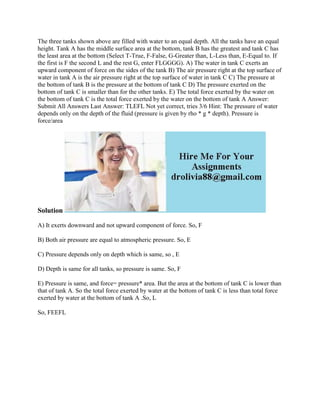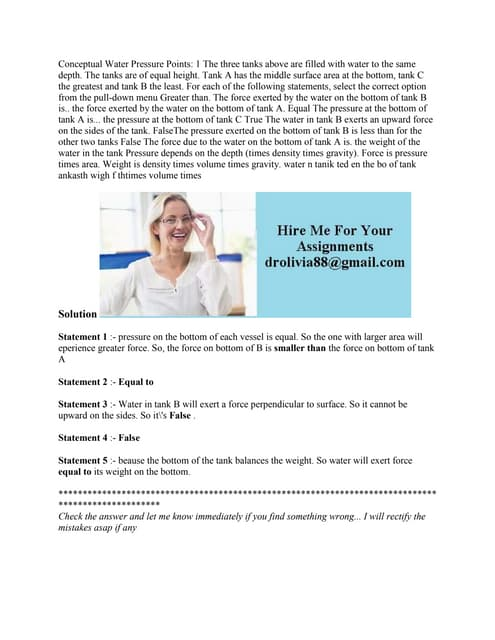AnúncioPróximos SlideSharesConceptual Water Pressure Points- 1 The three tanks above are filled w.docx
Carregando em ... 3
1 de 2
Anúncio

### The three tanks shown above are filled with water to an equal depth- A.docx

1. The three tanks shown above are filled with water to an equal depth. All the tanks have an equal height. Tank A has the middle surface area at the bottom, tank B has the greatest and tank C has the least area at the bottom (Select T-True, F-False, G-Greater than, L-Less than, E-Equal to. If the first is F the second L and the rest G, enter FLGGGG). A) The water in tank C exerts an upward component of force on the sides of the tank B) The air pressure right at the top surface of water in tank A is the air pressure right at the top surface of water in tank C C) The pressure at the bottom of tank B is the pressure at the bottom of tank C D) The pressure exerted on the bottom of tank C is smaller than for the other tanks. E) The total force exerted by the water on the bottom of tank C is the total force exerted by the water on the bottom of tank A Answer: Submit All Answers Last Answer: TLEFL Not yet correct, tries 3/6 Hint: The pressure of water depends only on the depth of the fluid (pressure is given by rho * g * depth). Pressure is force/area Solution A) It exerts downward and not upward component of force. So, F B) Both air pressure are equal to atmospheric pressure. So, E C) Pressure depends only on depth which is same, so , E D) Depth is same for all tanks, so pressure is same. So, F E) Pressure is same, and force= pressure* area. But the area at the bottom of tank C is lower than that of tank A. So the total force exerted by water at the bottom of tank C is less than total force exerted by water at the bottom of tank A .So, L So, FEEFL
Anúncio